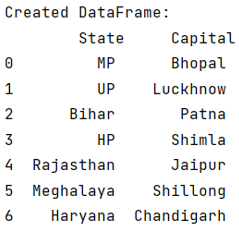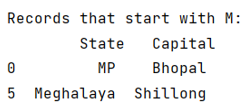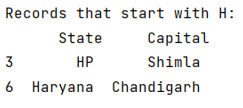# How to filter rows in pandas by regex?

Given a Pandas DataFrame, we have to filter rows by regex.
Submitted by Pranit Sharma, on June 02, 2022

Pandas is a special tool which allows us to perform complex manipulations of data effectively and efficiently. Inside pandas, we mostly deal with a dataset in the form of DataFrame. DataFrames are 2-dimensional data structure in pandas. DataFrames consists of rows, columns and the data.

## Problem statement

Here, we are going to learn how to filter rows in pandas using regex, regex or a regular expression is simply a group of characters or special characters which follows a particular pattern with the help of which we can search and filter pandas DataFrame rows.

## Regex (Regular Expression)

A special format string used for searching and filtering in pandas DataFrame rows.

### Example

• 'K.*': It will filter all the records which starts with the letter 'K'.
• 'A.*': It will filter all the records which starts with the letter 'A'.

As the regex is defined, we have to use the following piece of code for filtering DataFrame rows:

```dataframe.column_name.str.match(regex)
```
Note

To work with pandas, we need to import pandas package first, below is the syntax:

```import pandas as pd
```

Let us understand with the help of an example,

## Python code to create dataFrame

```# Importing pandas package
import pandas as pd

# Creating a Dictionary
d = {
"State": ["MP", "UP", "Bihar", "HP", "Rajasthan", "Meghalaya", "Haryana"],
"Capital": [
"Bhopal",
"Luckhnow",
"Patna",
"Shimla",
"Jaipur",
"Shillong",
"Chandigarh",
],
}

# Creating a DataFrame
df = pd.DataFrame(d)

# Display DataFrame
print("Created DataFrame:\n", df, "\n")
```

Output:Now, use regex filtration to filter DataFrame rows.

## Example 1: Python code to use regex filtration to filter DataFrame rows

```# Defining regex
regex = 'M.*'

# Here 'M.* means all the record that starts with M'

# Filtering rows
result = df[df.State.str.match(regex)]

# Display result
```

Output:## Example 2: Python code to use regex filtration to filter DataFrame rows

```# Defining regex
regex = 'H.*'

# Here 'H.* means all the record that starts with H'

# Filtering rows
result = df[df.State.str.match(regex)]

# Display result
```

Output: